Journal of Vibroengineering

Published: 30 June 2019

# Macroscopic and microscopic simulation of silo granular flow based on improved multi-element model

Yong Feng1
Ziran Yuan2
1, 2Henan University of Technology, Zhengzhou, China
Corresponding Author:
Ziran Yuan
Editor's Pick
Views 337

#### Abstract

In the PFC simulation of silo granular discharge, spherical particles were used in the traditional model, which could not accurately reflect macroscopic and mesoscopic mechanism during discharge of wheat, rice and other particles with non-spherical shapes. This research provides an improved multi-element model consisting of clump elements and ball elements. The model uses clump elements to simulate non-spherical grain particles and ball elements to simulate dust particles. The numerical simulation was carried out with the improved multi-element model, and the results are compared with the traditional simulation which uses the spherical ball elements and the experiment of grain discharge. It demonstrates that: (1) In terms of the normal wall pressure, the dynamic pressure fluctuation in flow with improved multi-element model is more gradual, and the discharge process lasts longer, the normal pressure simulation results are more accurate than the traditional model. (2) In terms of the meso-structure of the granular material, compared with traditional spherical ball model, the material packing porosity of the improved multi-element model decreases and the coordination number increases, which is denser and in consistent with the actual situation. (3) Particle shape would affect the meso-mechanical behavior of particles. The simulation results demonstrate that, compared with the traditional spherical ball model, the contact forces in the improved multi-element model increases, and the distribution of contact force chains is more uniform and denser; several arching force chains could be clearly seen in the improved multi-element model, which clearly reflects the dynamic change law of the instantaneous arch. The improved multi-element model established in this paper further improves the accuracy of simulation and reflects the dynamic changes of the normal pressure on the silo wall, granular material structure and meso-mechanical parameters during grain discharge.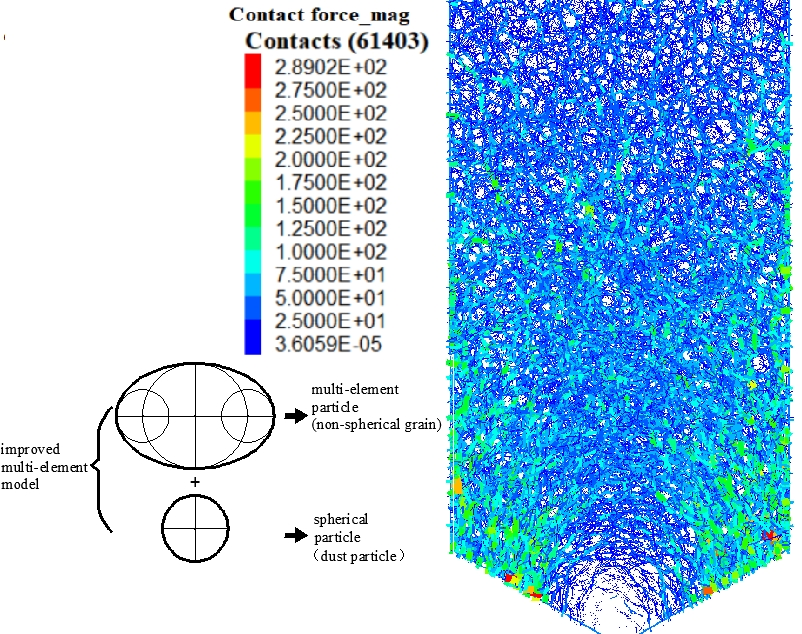#### Highlights

• An improved multi-element model is proposed to simulate the non-spherical particles and the dust contained in the material.
• The contact models between particles and walls are derived for the linear contact model, and the force-displacement update formula is established.
• Satisfactory results are obtained in simulation, and compare the results of the multi-element model and the traditional spherical ball model.

## 1. Introduction

Flow of granular materials in silos is important in many process engineering applications such as food, agricultural, and powder industries. The ﬂow behaviors of these granular materials are generally difficult to be predicted on account of the complex properties of granular materials [1, 2]. The comprehension of the ﬂow behaviors for non-spherical granular materials in the silo is important to the design and application of silos.

Since CUNDALL put forward the discrete element method, PFC, DEM and other numerical simulation software based on the discrete element method have been widely used in the field of granular materials research [3-5]. But most research focus on the spherical materials, the ball element in PFC is often used to simulate grain particles in silos [6-8]. However, there is significant deviation between macro-mechanical simulation and experimental results, and the meso-mechanical parameters of the particles are not comprehensive [9, 10]. An important reason for the above deviation is that, grain particles under natural conditions would not exist in a spherical shape [11, 12]. Furth more, the traditional spherical ball model cannot reflect the differences of non-spherical grain materials, such as wheat and paddy, and the influence of dust-containing rate on the discharge process. Therefore, it is difficult to accurately reflect the macroscopic and mesoscopic mechanical mechanism of grain flow in silos.

Some researchers have proposed clump element to simulate corn-shaped particles, disc-like particles and others [13, 14]. They indicate that the discrete element method is a powerful tool to analyze the interactions between irregularly shaped particles. Particle shape has a significant effect on the discharge rate, and particle interlocking might influence flow behavior in silos [15, 16]. However, in previous work, there is no quantitative study on the effect of grain shape and dust content, the accuracy of macro parameters suck as wall pressure in flow is not studied enough, and the mesoscopic mechanism studied in previous work are not comprehensive enough. In addition, the range of deviations in the case of non-spherical materials such as wheat-shaped particles in flow is unknown. The mesoscopic simulation results such as packing porosity, the coordination number and the dynamic force chains were examined and compared with the experiment result in the literature. This paper provided more comprehensive quantification and analysis.

Based on the above analysis, an improved multi-element model consisting of clump elements and ball elements is proposed in this paper. The model would use clump elements to simulate irregular grain particles [17, 18] and small ball elements to simulate dust particles. On the basis of deducing the contact constitutive relation in particles, between particles and environment [19-21]. The numerical simulation with the improved multi-element model was carried out based on the experiment of grain discharge, and the simulation results of improved model were compared with the traditional spherical ball model.

The current work focuses to establish an improved multi-element model for non-spherical grain particles such as wheat and paddy, to simulate the effects of grain morphology and dust content on the macroscopic and mesoscopic mechanical parameters during discharge, to reveal the macroscopic and mesoscopic mechanical mechanism objectively and accurately and provide a reference for the numerical simulation of irregular grain. In the field of engineering application, the improved model could be used to simulate and predict the wall pressure of silos and provide guidance for silo design.

## 2. Experiment test

The model is made according to the actual common silos reasonable size of the scale 20:1. The height of the experimental silo is 1000mm, the radius of silo is 250 mm, the wall thickness is 5 mm, and the half angle of the hopper part is 60°. The strain gauge is arranged at each monitoring points of the silo cylinder wall, to detect the normal pressure of the silo cylinder part during discharge. The geometry of the silo model and the arrangement of monitoring points are shown in Fig. 1. The experimental model is shown in Fig. 2. The model silo was filled with wheat, diameters ranging from 5 mm to 6 mm, and the orifice plate would be removed for discharge after filling and store for two days.

Fig. 1Silo model geometry and monitoring points arrangement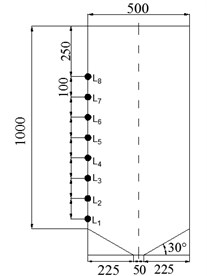Fig. 2Grain discharge test system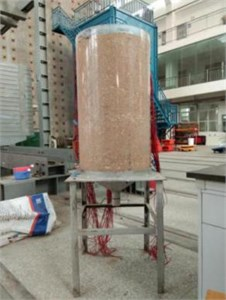## 3. Numerical models

### 3.1. Improved multi-element model

The improved multi-elements model proposed in this paper consists of clump elements and small ball elements. The model would use multi-elements to simulate non-spherical grain particles, and use small ball elements to simulate dust particles. Multi-element particles would simulate the non-spherical particles with “clump”, which consists of three rigid spheres overlapping with each other. This research focus on the shape of non-spherical grain particles (such as wheat, rice), and the improved multi-element model system is shown in Fig. 3.

Fig. 3Improved multi-element model system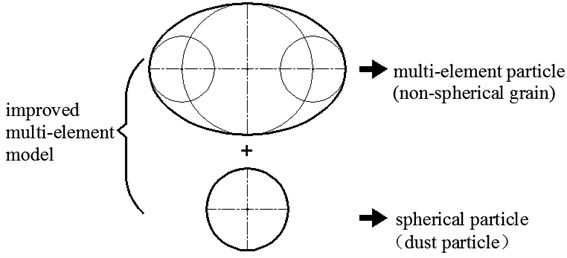### 3.2. Contact model

The recent research demonstrates that linear contact model is widely used in PFC simulation in the granular flow [22-24], the contact models between particles and walls are derived respectively for the numerical simulation, and the force-displacement update formula is established, which is described in this section.

### 3.2.1. Contact between particles

1. Multi-element particles.

The multi-element particle is simplified as an ellipse. According to the force-displacement law and the calculation method in simulation [25, 26], the unit vector model and the contact deformation of two particles could be obtained. Two elliptical particles are in contact with each other as shown in Fig. 4, and it is considered that the normal and the tangential vector of particles at the contact point is:

1
$\stackrel{\to }{\mathbf{n}}=\left({n}_{x},{n}_{y}\right),$
2
$\stackrel{\to }{\mathbf{t}}=\left({t}_{x},{t}_{y}\right).$

Fig. 4Contact between multi-element particles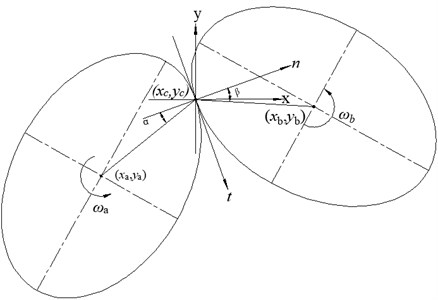According to the geometrical meaning of ellipse, the radius vector of ellipse $\stackrel{\to }{{\mathbf{r}}_{a}}$, $\stackrel{\to }{{\mathbf{r}}_{b}}$ and the angle between the normal vector are $\alpha$ and $\beta$, respectively. The center coordinates of the particles are respectively, and where $\stackrel{\to }{{\mathbf{v}}_{a}}$, $\stackrel{\to }{{\mathbf{v}}_{b}}$ is the velocity of the particles’ centers. The velocity of two particles is expressed as Eqs. (3) and (4) at the contact point:

3
$\stackrel{\to }{{\mathbf{v}}_{ca}}=\stackrel{\to }{{\mathbf{v}}_{a}}+\stackrel{\to }{{\mathbf{w}}_{a}}×\stackrel{\to }{{\mathbf{r}}_{a}},$
4
$\stackrel{\to }{{\mathbf{v}}_{cb}}=\stackrel{\to }{{\mathbf{v}}_{b}}+\stackrel{\to }{{\mathbf{w}}_{b}}×\stackrel{\to }{{\mathbf{r}}_{b}}.$

The relative velocity at the contact point of the two particles is Eqs. (5) and (6):

5
$\stackrel{\to }{\mathbf{d}{\mathbf{v}}_{c}}=\stackrel{\to }{{\mathbf{v}}_{ca}}-\stackrel{\to }{{\mathbf{v}}_{cb}},$
6
$\stackrel{\to }{\mathbf{d}{\mathbf{v}}_{c}}=\stackrel{\to }{{\mathbf{v}}_{a}}+\stackrel{\to }{{\mathbf{w}}_{a}}×\stackrel{\to }{{\mathbf{r}}_{a}}-\left(\stackrel{\to }{{\mathbf{v}}_{b}}+\stackrel{\to }{{\mathbf{w}}_{b}}×\stackrel{\to }{{\mathbf{r}}_{b}}\right).$

The component relative velocity in the normal and the tangential directions is defined as Eqs. (7) and (8) respectively:

7
$\stackrel{\to }{\mathbf{d}{\mathbf{v}}_{n}}=\left(\stackrel{\to }{{\mathbf{v}}_{a}}-\stackrel{\to }{{\mathbf{v}}_{b}}\right)\stackrel{\to }{\mathbf{n}}+\left(\stackrel{\to }{{\mathbf{w}}_{a}}×\stackrel{\to }{{\mathbf{r}}_{a}}\mathrm{s}\mathrm{i}\mathrm{n}\alpha -\stackrel{\to }{{\mathbf{w}}_{b}}×\stackrel{\to }{{\mathbf{r}}_{b}}\mathrm{s}\mathrm{i}\mathrm{n}\beta \right),$
8
$\stackrel{\to }{\mathbf{d}{\mathbf{v}}_{t}}=\left(\stackrel{\to }{{\mathbf{v}}_{a}}-\stackrel{\to }{{\mathbf{v}}_{b}}\right)\stackrel{\to }{\mathbf{t}}+\left(\stackrel{\to }{{\mathbf{w}}_{a}}×\stackrel{\to }{{\mathbf{r}}_{a}}\mathrm{c}\mathrm{o}\mathrm{s}\alpha -\stackrel{\to }{{\mathbf{w}}_{b}}×\stackrel{\to }{{\mathbf{r}}_{b}}\mathrm{c}\mathrm{o}\mathrm{s}\beta \right).$

2. Multi-element particle and spherical particle.

Multi-element particle and spherical particle contact with each other (Fig. 5), the center coordinates of the spherical particle $a$ is $\left({x}_{a},{y}_{a}\right)$, the center coordinates of the ellipse particle $b$ is $\left({x}_{b},{y}_{b}\right)$, and the angle between the radius vector of ellipse center and the normal vector $\stackrel{\to }{\mathbf{n}}$ at the contact point is $\beta$. The velocity of particles $a$, $b$ are defined as $\stackrel{\to }{{\mathbf{v}}_{a}}$, $\stackrel{\to }{{\mathbf{v}}_{b}}$, where the velocity of two particles and the relative velocity of the two particles at the contact points expressed with reference to the contact between two multi-element particles. The relative velocity is Eq. (9):

9
$\stackrel{\to }{\mathbf{d}{\mathbf{v}}_{c}}=\left(\stackrel{\to }{{\mathbf{v}}_{a}}-\stackrel{\to }{{\mathbf{v}}_{b}}\right)+\left(\stackrel{\to }{{\mathbf{w}}_{a}}×\stackrel{\to }{{\mathbf{r}}_{a}}-\stackrel{\to }{{\mathbf{w}}_{b}}×\stackrel{\to }{{\mathbf{r}}_{b}}\right).$

The component relative velocity in the normal and the tangential directions is calculated as Eqs. (10) and (11) respectively:

10
$\stackrel{\to }{\mathbf{d}{\mathbf{v}}_{n}}=\left(\stackrel{\to }{{\mathbf{v}}_{a}}-\stackrel{\to }{{\mathbf{v}}_{b}}\right)\stackrel{\to }{\mathbf{n}}-\stackrel{\to }{{\mathbf{w}}_{b}}×\stackrel{\to }{{\mathbf{r}}_{b}}\mathrm{s}\mathrm{i}\mathrm{n}\beta ,$
11
$\stackrel{\to }{\mathbf{d}{\mathbf{v}}_{t}}=\left(\stackrel{\to }{{\mathbf{v}}_{a}}-\stackrel{\to }{{\mathbf{v}}_{b}}\right)\stackrel{\to }{\mathbf{t}}+\stackrel{\to }{{\mathbf{w}}_{a}}×\stackrel{\to }{{\mathbf{r}}_{a}}-\stackrel{\to }{{\mathbf{w}}_{b}}×\stackrel{\to }{{\mathbf{r}}_{b}}\mathrm{c}\mathrm{o}\mathrm{s}\beta .$

Fig. 5Contact between multi-element particle and spherical particle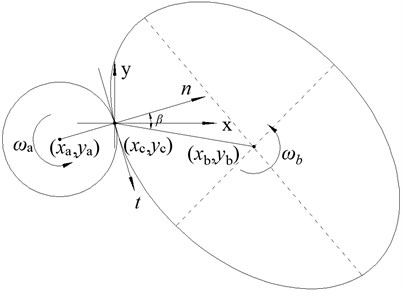3. Spherical particles.

The mesoscopic state of the two spherical particles, which contact with each other as shown in Fig. 6, and the center coordinates of the two particles are $\left({x}_{a},{y}_{a}\right)$, $\left({x}_{b},{y}_{b}\right)$, $\stackrel{\to }{\mathbf{r}}$ is the radius vector of two particles and the central velocity of the spherical particles are defined as $\stackrel{\to }{{\mathbf{v}}_{a}}$, $\stackrel{\to }{{\mathbf{v}}_{b}}$ respectively. The velocity of two particles at the contact point is expressed with reference as mentioned, the relative velocity of the two particles at the contact point is Eq. (12):

12
$\stackrel{\to }{\mathbf{d}{\mathbf{v}}_{c}}=\left(\stackrel{\to }{{\mathbf{v}}_{a}}-\stackrel{\to }{{\mathbf{v}}_{b}}\right)+\left(\stackrel{\to }{{\mathbf{w}}_{a}}×\stackrel{\to }{\mathbf{r}}-\stackrel{\to }{{\mathbf{w}}_{b}}×\stackrel{\to }{\mathbf{r}}\right).$

Fig. 6Contact between spherical particles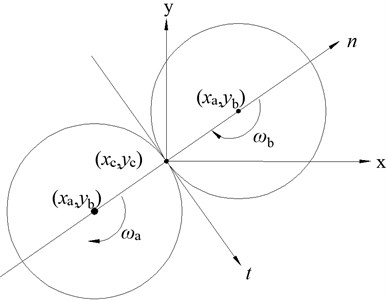The component relative velocity in the normal and the tangential directions is calculated as Eqs. (13) and (14) respectively:

13
$\stackrel{\to }{\mathbf{d}{\mathbf{v}}_{n}}=\stackrel{\to }{\mathbf{d}{\mathbf{v}}_{c}}\stackrel{\to }{\mathbf{n}}=\left(\stackrel{\to }{{\mathbf{v}}_{a}}-\stackrel{\to }{{\mathbf{v}}_{b}}\right)\stackrel{\to }{\mathbf{n}},$
14

### 3.2.2. Contact between particle and wall

The wall, which defined as b, rotate around the point $\left({x}_{b},{y}_{b}\right)$, particle is defined as a and the center coordinate of the particle is $\left({x}_{a},{y}_{a}\right)$. The coordinates of the contact point between the wall and the particle is $\left({x}_{c},{y}_{c}\right)$. The normal and tangential vectors at the contact point are $\stackrel{\to }{\mathbf{n}}=\left({n}_{x},{n}_{y}\right)$, $\stackrel{\to }{\mathbf{t}}=\left({t}_{x},{t}_{y}\right)$. The $\stackrel{\to }{{\mathbf{v}}_{a}}$, $\stackrel{\to }{{\mathbf{v}}_{b}}$ are the velocity of the particle and the wall. The wall would not translate, so the translate velocity of the wall $\stackrel{\to }{{\mathbf{v}}_{b}}$ is zero, where ${w}_{b}$ is the angular velocity of the wall rotation. Where $\stackrel{\to }{{\mathbf{r}}_{w}}$ is the vector from $\left({x}_{b},{y}_{b}\right)$ to the contact point $\left({x}_{c},{y}_{c}\right)$.

1. Multi-element particle and wall.

Fig. 7 is the schematic diagram of multi-element particle in contact with the wall. The velocity of particle and wall is calculated as Eqs. (15) and (16), the relative velocity at the contact point is Eq. (17):

15
$\stackrel{\to }{{\mathbf{v}}_{ca}}=\stackrel{\to }{{\mathbf{v}}_{a}}+\stackrel{\to }{{\mathbf{w}}_{a}}×\stackrel{\to }{{\mathbf{r}}_{a}},$
16
$\stackrel{\to }{{\mathbf{v}}_{cb}}=\stackrel{\to }{{\mathbf{w}}_{b}}×\stackrel{\to }{{\mathbf{r}}_{w}},$
17
$\stackrel{\to }{\mathbf{d}{\mathbf{v}}_{c}}=\stackrel{\to }{{\mathbf{v}}_{a}}+\left(\stackrel{\to }{{\mathbf{w}}_{a}}×\stackrel{\to }{{\mathbf{r}}_{a}}-\stackrel{\to }{{\mathbf{w}}_{b}}×\stackrel{\to }{{\mathbf{r}}_{w}}\right).$

Fig. 7Contact between wall and multi-element particle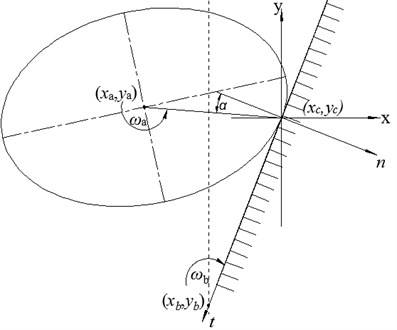The component relative velocity is defined in the normal and tangential directions as follows:

18
$\stackrel{\to }{\mathbf{d}{\mathbf{v}}_{n}}=\stackrel{\to }{\mathbf{d}{\mathbf{v}}_{c}}\stackrel{\to }{\mathbf{n}}=\stackrel{\to }{{\mathbf{v}}_{a}}\stackrel{\to }{\mathbf{n}}-\stackrel{\to }{{\mathbf{w}}_{a}}×\stackrel{\to }{{\mathbf{r}}_{a}}\cdot \mathrm{s}\mathrm{i}\mathrm{n}\alpha +\stackrel{\to }{{\mathbf{w}}_{b}}×\stackrel{\to }{{\mathbf{r}}_{w}},$
19
$\stackrel{\to }{\mathbf{d}{\mathbf{v}}_{t}}=\stackrel{\to }{\mathbf{d}{\mathbf{v}}_{c}}\stackrel{\to }{\mathbf{t}}=\stackrel{\to }{{\mathbf{v}}_{a}}\stackrel{\to }{\mathbf{t}}-\stackrel{\to }{{\mathbf{w}}_{a}}×\stackrel{\to }{{\mathbf{r}}_{a}}\cdot \mathrm{c}\mathrm{o}\mathrm{s}\alpha .$

2. Spherical particle and wall.

The velocity expression of particle “$a$” and wall “$b$” at the contact point is similar to that of multi-element and wall. The relative velocity is defined in the normal and the tangential directions as follows:

20
$\stackrel{\to }{\mathbf{d}{\mathbf{v}}_{cn}}=\stackrel{\to }{\mathbf{d}{\mathbf{v}}_{c}}\stackrel{\to }{\mathbf{n}}=\stackrel{\to }{{\mathbf{v}}_{a}}\stackrel{\to }{\mathbf{n}}+\stackrel{\to }{{\mathbf{w}}_{b}}×\stackrel{\to }{{\mathbf{r}}_{w}}\stackrel{\to }{\mathbf{n}},$
21
$\stackrel{\to }{\mathbf{d}{\mathbf{v}}_{ct}}=\stackrel{\to }{\mathbf{d}{\mathbf{v}}_{c}}\stackrel{\to }{\mathbf{t}}=\stackrel{\to }{{\mathbf{v}}_{a}}\stackrel{\to }{\mathbf{t}}+\stackrel{\to }{{\mathbf{w}}_{a}}×\stackrel{\to }{\mathbf{r}}\stackrel{\to }{\mathbf{t}}.$

Fig. 8Contact between wall and spherical particle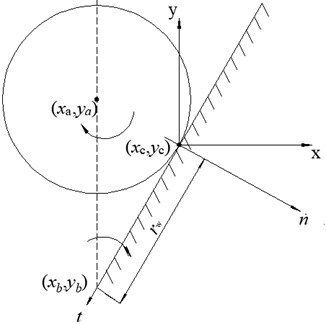### 3.3. Equations of particle motion

The normal and the tangential displacement of particles and walls would generate by the velocity component in the corresponding direction [27, 28], we could obtain the displacement component Eqs. (22) and (23), the displacement would change in a period time according to the velocity component calculated by the above mentioned:

22
$\stackrel{\to }{\mathrm{\Delta }{\mathbf{r}}_{n}}=\stackrel{\to }{\mathbf{d}{\mathbf{v}}_{n}}\mathrm{\Delta }t,$
23
$\stackrel{\to }{\mathrm{\Delta }{\mathbf{r}}_{t}}=\stackrel{\to }{\mathbf{d}{\mathbf{v}}_{t}}\mathrm{\Delta }t.$

The calculation method of force in PFC linear model is:

24
$\stackrel{\to }{\mathrm{\Delta }{\mathbf{F}}_{n}}={k}_{n}\stackrel{\to }{\mathrm{\Delta }{\mathbf{r}}_{n}},$
25
$\stackrel{\to }{\mathrm{\Delta }{\mathbf{F}}_{t}}={k}_{t}\stackrel{\to }{\mathrm{\Delta }{\mathbf{r}}_{t}}.$

The equation of motion of particles according to Newton’s second law is as follows:

26
$\sum \stackrel{\to }{\mathbf{F}}=m\frac{\stackrel{\to }{\mathbf{d}\mathbf{v}}}{dt}=m\frac{{\stackrel{\to }{\mathbf{d}\mathbf{r}}}^{2}}{{d}^{2}t},$
27
$\sum \stackrel{\to }{\mathbf{M}}=I\frac{\stackrel{\to }{\mathbf{d}\mathbf{w}}}{dt}=I\frac{{\stackrel{\to }{\mathbf{d}\mathbf{\theta }}}^{2}}{{d}^{2}t},$

where $I$ is the inertia moment of the particle, in the linear contact model, it is generally considered that the moment at the contact point is zero, where $\sum \stackrel{\to }{\mathbf{F}}$ is subjected to the combined force of contact force and gravity.

The mesoscopic parameters are updated in each step of simulation. For example, particle a, the force is calculated as:

28
$\stackrel{\to }{{\mathbf{F}}_{n}\left(t+\mathrm{\Delta }t\right)}=\stackrel{\to }{{\mathbf{F}}_{n}\left(t\right)}+\stackrel{\to }{\mathrm{\Delta }{\mathbf{F}}_{n}\left(t\right)},$
29
$\stackrel{\to }{{\mathbf{F}}_{t}\left(t+\mathrm{\Delta }t\right)}=\stackrel{\to }{{\mathbf{F}}_{t}\left(t\right)}+\stackrel{\to }{\mathrm{\Delta }{\mathbf{F}}_{t}\left(t\right)}.$

The velocity is updated as:

30
$\stackrel{\to }{{\mathbf{v}}_{a}\left(t+\mathrm{\Delta }t\right)}=\stackrel{\to }{{\mathbf{v}}_{a}\left(t\right)}+\frac{\sum \stackrel{\to }{\mathbf{F}}}{m}\mathrm{\Delta }t,$
31
$\stackrel{\to }{{\mathbf{w}}_{a}\left(t+\mathrm{\Delta }t\right)}=\stackrel{\to }{{\mathbf{w}}_{a}\left(t\right)}+\frac{\sum \stackrel{\to }{\mathbf{M}}}{I}\mathrm{\Delta }t.$

The particle position and rotation angle is updated as:

32
$\stackrel{\to }{{\mathbf{r}}_{a}\left(t+\mathrm{\Delta }t\right)}=\stackrel{\to }{{\mathbf{r}}_{a}\left(t\right)}+\stackrel{\to }{{\mathbf{v}}_{a}\left(t+\frac{\mathrm{\Delta }t}{2}\right)}\mathrm{\Delta }t,$
33
$\stackrel{\to }{{\mathbf{\theta }}_{a}\left(t+\mathrm{\Delta }t\right)}=\stackrel{\to }{{\mathbf{\theta }}_{a}\left(t\right)}+\stackrel{\to }{{\mathbf{v}}_{a}\left(t+\frac{\mathrm{\Delta }t}{2}\right)}\mathrm{\Delta }t.$

### 3.4. Discrete element model

The silo model with a radius of 250 mm and a height of 1000 mm is established according to the geometry of experimental silo. The wall element would be used to simulate the silo wall. The multi-element particles with 6 mm major axis diameter and 4 mm minor axis diameter would be used to simulate non-spherical particles. The dust particles are simulated by ball elements with 0.5 mm diameter, the dust particles added in model with a ratio of 10:1. (The traditional model would only use the spherical particles with diameter of 5 mm). The volume of ball element used in the traditional ball model is 19.63, and that of multi-element particle in the improved model is 18.84, which is 4.02 % lower than the traditional model. The ten sections of walls would be built on both sides according to the arrangement of monitoring points in the experiment. The normal pressure of each wall section is established to monitor and record the dynamic pressure, which makes the same effect as the monitoring points in the experiment.

The parameters are selected with the laboratory measurement and the mechanical properties of grain [29-31]. Through the parameter calibration method [32-34], the physical and mechanical parameters is adjusted to achieve that, the static storage normal pressure in simulation is in accordance with that measured in experiment. The parameters determined are listed in Table 1. In Table 1, the wall, ball and clump elements represent the wall, spherical particles and non-spherical grain particles, respectively, the parameters selected for the mechanical contact model of wall and particle are listed in Table 2, and ${k}_{n}$, ${k}_{s}$ are defined as their normal and tangential stiffness.

Table 1The main physical parameters in the simulation

 Parameter category Numerical value Spherical ball model Ball density / (kg·m-3) 1000 Ball friction coefficient 0.43 Wall friction coefficient 0.62 Particle damp coefficient 0.05 Improved multi-element model Ball density / (kg·m-3) 2000 Clump density / (kg·m-3) 1000 Ball friction coefficient 0.3 Clump friction coefficient 0.3 Wall friction coefficient 0.62 Particle damp coefficient 0.05

Table 2Mechanics parameters of particle and wall in simulation

 Category Numerical value Spherical ball model Wall-kn / (N·m-1) 3.2e6 Wall-ks / (N·m-1) 1.6e6 Ball-kn / (N·m-1) 3.2e5 Ball-ks / (N·m-1) 1.6e5 Improved multi-element model Wall-kn / (N·m-1) 3.2e6 Wall-ks / (N·m-1) 1.6e6 Clump-kn / (N·m-1) 3.2e5 Clump-ks / (N·m-1) 1.6e5 Ball-kn / (N·m-1) 3.2e6 Ball-ks / (N·m-1) 1.6e6

## 4. Results and discussion

### 4.1. Fill simulation

The traditional spherical particles or the improved multi-element particles would fill into the silo by the method of central fill with properties selected according to the calculation ability of computer and the parameter calibration method mentioned above. The particles would operate just as the linear contact model, which calculate as the contact model and the update method in Chapter 2.

Because the filling process would be a long computing time and the calculation would be complicated if the particles internal friction coefficient is large, so a new filling method is adopted, which the particles internal friction coefficient is set to zero during filling. The particles internal friction coefficient is listed in Table 1 and Table 2 by “the ball property (clump property)” command after the filling process is completed, then iterate for a certain period of time to achieve stability (Fig. 9).

The traditional spherical particles model contains 23,000 ball elements and the storage height is 0.994 m. The improved multi-element particles model contains 24676 clump elements and 2382 small ball elements with a storage height of 1.013. The scale of the two models is similar.

Fig. 9Static storage condition: a) traditional spherical particles model, b) improved multi-element model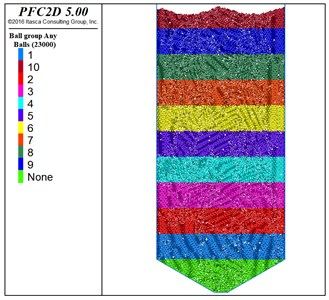a)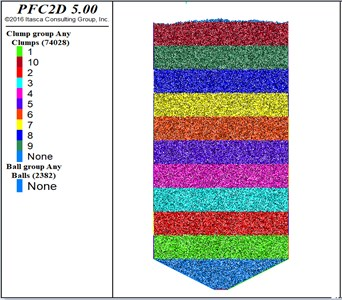b)

### 4.2. Simulation & experiment

Because this research focus on the grain discharge process of the cylindrical silos, it is unnecessary to determine the flow pattern is the mass flow or the funnel flow. Therefore, this paper uses the pressure to verify whether the simulation is consistent with the experiment. Comparing the normal pressure of silo wall in storage condition with the pressure obtained by the experiment in the Chapter 2, the results demonstrate that the results from simulation, experiment and the trend of pressure match well, which can verify the authenticity of the simulation model. The comparison of dynamic pressure in the following further validated the proposed simulation model.

Fig. 10Static storage wall pressure value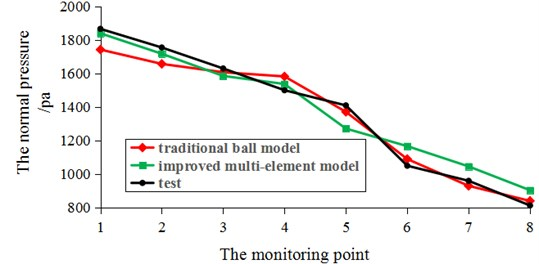### 4.3.1. Normal wall pressure

Since the pressure at the monitoring points of the silo upper part is small and after a period of discharge the pressure of the upper part monitoring points would be zero, which would introduce observation difficult, only three monitoring points (1.3.5) were selected to analyze the dynamic normal pressure in grain discharge (Fig. 11).

Fig. 11Dynamic pressure simulation results: a) the traditional spherical ball model, b) the improved multi-element particle model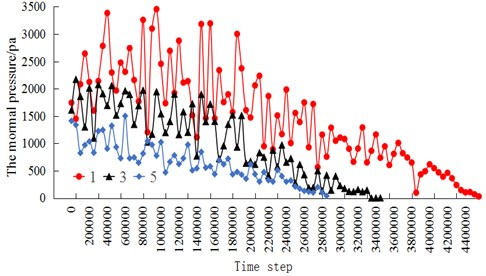a)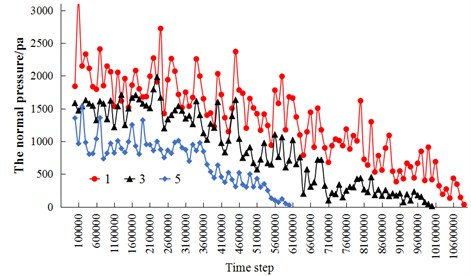b)

It is demonstrated that, the dynamic pressure decreases with the decreases of depth in two models, which is consistent with the results provided in [35-37]. The traditional spherical ball model iterated 4500000 steps to discharge, the real time of discharge is 29.1 s, while the improved multi-element model iterates 10800000 steps to discharge, and the real time is 31.4 s. It indicates that the discharge time of improved multi-element model is longer and the outflow is more stagnant.

Taking the No. 1 monitoring point for analysis, the dynamic normal pressure during discharge calculated by the traditional spherical ball model and the improved multi-element model is compared with the experimental results (Fig. 12).

Fig. 12Comparison of dynamic pressure during discharge process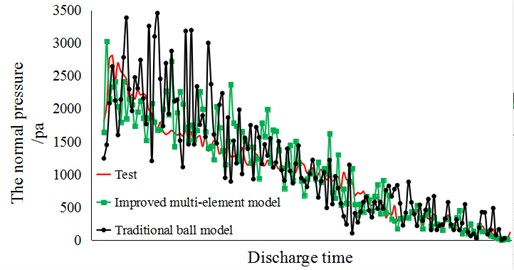It is demonstrated that, the pressure of simulation models fluctuates more volatile, but the dynamic pressure trend is similar; the simulation values fluctuate around the experimental values. The other monitoring points illustrate similar laws, which can further verify the authenticity of the simulation model. The pressure fluctuation amplitude of the improved multi-element model is smaller, and the fluctuation trend is gentler, which is related to the inter-locking between particles, and the inter-friction coefficient of the improved multi-element model is more sufficient than that of the traditional ball model in flow.

The error of simulation could be calculated as:

34
$W=\frac{{\sum }_{1}^{n}\sqrt{\left({p}_{A}-{p}_{B}{\right)}^{2}/{{p}_{B}}^{2}}}{n},$

where ${p}_{A}$ is defined as the simulation pressure of improved multi-element model or the traditional spherical ball model, ${p}_{B}$ is the experimental results. It is calculated that the deviation of the improved multi-element model is 11.28 %, while that of the traditional model is 29.175 %, it demonstrates that the improved multi-element model effectively improve the accuracy of dynamic pressure during discharge.

Table 3Dynamic maximum pressure value comparison

 Monitor points Test Spherical ball model Deviation Improved model Deviation 1 2845.540 3455.140 21.44 % 3027.398 6.39 % 3 1906.340 2170.029 13.85 % 1982.010 3.98 % 5 1679.017 1501.752 10.63 % 1531.333 8.81 % 7 1056.897 1232.434 16.74 % 1123.435 6.34 %

### 4.3.2. Porosity and coordination number

For the improved multi-element model, the “measure circle” command provided by PFC is used to establish the measure circle in the silo (Fig. 13). Comparing the meso-structural parameters of the improved multi-element model and the traditional ball model, the meso-mechanical behaviors of the two models would be analyzed as follows.

Fig. 13The position of measure circles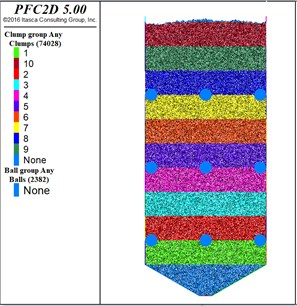The method for determining porosity as Eq. (35) could be inferred from the definition of porosity . Where $V$ is the grain accumulation volume, ${V}_{p}$ is the sum volume of stored grain particles:

35
$n=\frac{V-{V}_{p}}{V}.$

The porosity of the laboratory test results is 40.04 % in the static storage state. The average porosity of nine measure circles in the 2D model, which is transformed from the porosity :

36
${\epsilon }_{3d}=1-\xi \left(1-{\epsilon }_{2d}\right),$
37
$\xi =\frac{2}{\sqrt{\pi \sqrt{3}}}+{D}_{r}\left(\frac{2}{\sqrt{\pi \sqrt{3}}}-\frac{\sqrt{2}}{\sqrt{\pi \sqrt{3}}}\right),$

where ${D}_{r}$ is the relative compaction of grain, ${D}_{r}=\frac{{e}_{max}-e}{{e}_{max}-{e}_{min}}$, ${e}_{max}$, ${e}_{min}$, $e$ are the maximum, the minimum and the test void ratios in laboratory tests, respectively. ${\epsilon }_{2d}$ is two-dimensional porosity of grain, ${\epsilon }_{3d}$ is three-dimensional porosity of grain.

It is calculated that the porosity of the improved multi-element model is 39.13 % and the error is 2.27 % in the three-dimensional state, while the porosity of the spherical ball model is 42.20 % and the difference is 5.39 %. The packing porosity decreased by 3.07 % and the accuracy of the improved model is increased by 3.12 % in simulation.

The coordination number is also one of the important meso-structural parameters, which would be used to characterize the compactness of the accumulated particles like porosity. The coordination number is defined as the number of particles in contact with a certain particle, and the coordination number is inversely proportional to the porosity . The coordination number of the traditional ball model is 3.67 and that of the improved multi-element model is 4.66, which indicates that the improved multi-element model could effectively increase the coordination number of the particle assemble. In terms of meso-structural parameters, the improved multi-element model makes the grain denser and more in accord with the actual situation of grain storage .

### 4.3.3. Dynamic force chain

The dynamic changes of the meso-mechanics were analyzed by comparing the force chain diagrams of the two models at the mechanical time of 2.4 s (Fig. 14).

Fig. 14The diagram of dynamic force chains: a) traditional spherical ball model, b) improved multi-element particle model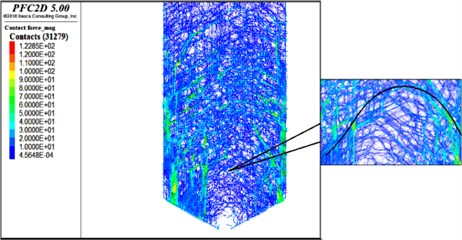a)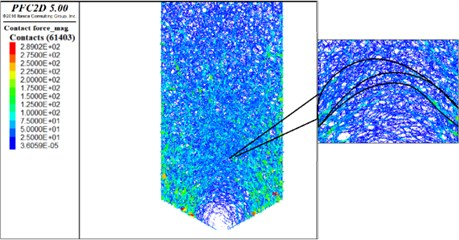b)

The substantial particle chains are easily formed between multi-element particles to resist external forces because of the inter-locking between particles [42-44].

It could be clearly found that the number of contact forces of the traditional spherical ball model is 31279, and that of the improved multi-element model is 61403. It indicates that the force chain of the improved multi-element model is denser, and the force network is more complex. As shown in the local force chain diagram of the two models (Fig. 15), the contact force distribution of the improved multi-element model is more uniform. There are several transverse arching force chains observed clearly in the improved multi-element model. The arch occurs frequently during discharge . The simulation results of multi-element model could more clearly reflect the law of instantaneous arch which.

## 5. Conclusions

The PFC simulation was developed to simulate the non-spherical particles discharge with multi-element model considering the effect of dust content with ball elements. The numerical simulation was carried out by using the improved multi-element model on the basis of the experiment, and the simulation results of improved multi-element model were compared with those of the traditional spherical ball model. The research indicated that, the particle shape not only affects the macro-mechanical behavior during flow, but also affects the meso-structure of particles and the dynamic force chains evolution in flow. The specific conclusions are as follows:

1) In terms of macroscopic wall pressure, the dynamic pressure fluctuation during discharge simulated with improved multi-element particle model is more gradual, and the discharge process lasts longer. The error, which by comparing the results of dynamic pressure obtained from simulation and experiment, of the improved multi-element particle model is 11.28 %, while the error of the traditional spherical model is 29.175 %. The error of the improved multi-element model is 17.895 % lower than that of the traditional spherical ball model. The normal pressure simulation results are more accurate than the traditional ball model.

2) In terms of the meso-structure of the granular material, the packing porosity of the traditional spherical particle model is 42.20 %, the coordination number is 3.67, while the material packing porosity of the improved model is 39.13 %, the coordination number is 4.66. And the packing porosity decrease by 3.07 %, the accuracy of the improved model increased by 3.12 %, and the coordination number increase by 0.99. The improved multi-element model can effectively increase the density of particle accumulation, and more in consistent with the actual situation.

3) The particle shape affects the meso-mechanical behavior of particles. The simulation demonstrates that, compared with the traditional spherical ball model, the number of contact forces in the improved multi-element model increases by 30124, the distribution of contact force chains is more uniform and dense, and several arching force chains could be clearly observed in the improved multi-element model, which more clearly reflect the law of the instantaneous arch.

This research proved that the particle shape has a significant effect on the macroscopic and mesoscopic porosities of granular materials. The improved multi-element particles established in this paper would further improve the accuracy of simulation; the comprehension of the ﬂow behaviors for non-spherical granular materials in the silo is important to the design and application of silos. The research has an important reference value for further simulation of non-spherical grain particles’ flow mechanism in the silo. In the field of engineering application, the improved model could be used to simulate and predict the wall pressure of silos and provide guidance for silo design.

#### Acknowledgements

Our deepest gratitude goes first and foremost to the National Science Foundation Project of China 51708182 for their fund support.